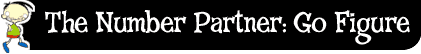﻿ The number partner: go figure: Counting on# Counting on

Counting on can help you work out number pairs . It is easier to count on from the larger number of a number pair.

For example, let's look at the number 9.

To break up the number 9, I would think of a number smaller than 9, like 5. Then I would count on, using my fingers, from 5 until I got to 9 (6, 7, 8, 9) This would use 4 fingers.

5 plus 4 equals 9.

5 and 4 is a number pair.

4 and 5 is the same number pair.

Now let's look at the number 18.

Can you count on from 12 to work out a number pair for 18?

Did you count on 6?

12 plus 6 equals 18.

This is the same as 6 plus 12 equals 18.